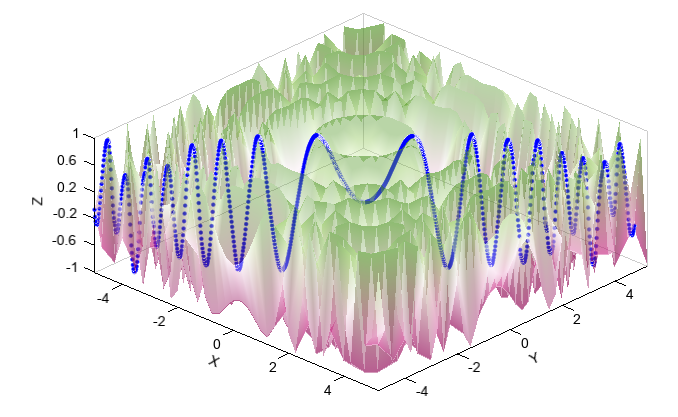# interp2d¶

class numeric.interpolate.interp2d(object)

Interpolate over a 2-D grid.

x, y and z are arrays of values used to approximate some function f: z = f(x, y). This class returns a function whose call method uses spline interpolation to find the value of new points.

If x and y represent a regular grid, consider using RectBivariateSpline.

Parameters
• x – (array_like) 1-D arrays of x coordinate in strictly ascending order.

• y – (array_like) 1-D arrays of y coordinate in strictly ascending order.

• z – (array_like) 2-D array of data with shape (x.size,y.size).

• kind – (boolean) Specifies the kind of interpolation as a string (‘linear’, ‘nearest’). Default is ‘linear’.

Examples:

x = np.arange(-5.01, 5.25, 0.25)
y = np.arange(-5.01, 5.25, 0.25)
xx, yy = np.meshgrid(x, y)
z = np.sin(xx**2+yy**2)
f = interpolate.interp2d(x, y, z, kind='spline')

xnew = np.arange(-5.01, 5.01, 1e-2)
ynew = np.arange(-5.01, 5.01, 1e-2)
znew = f(xnew, ynew)

scatter3(xnew, ynew, znew, 4, c='b')
surf(xx, yy, z, edgecolor=None, cmap='MPL_PiYG', alpha=0.4)